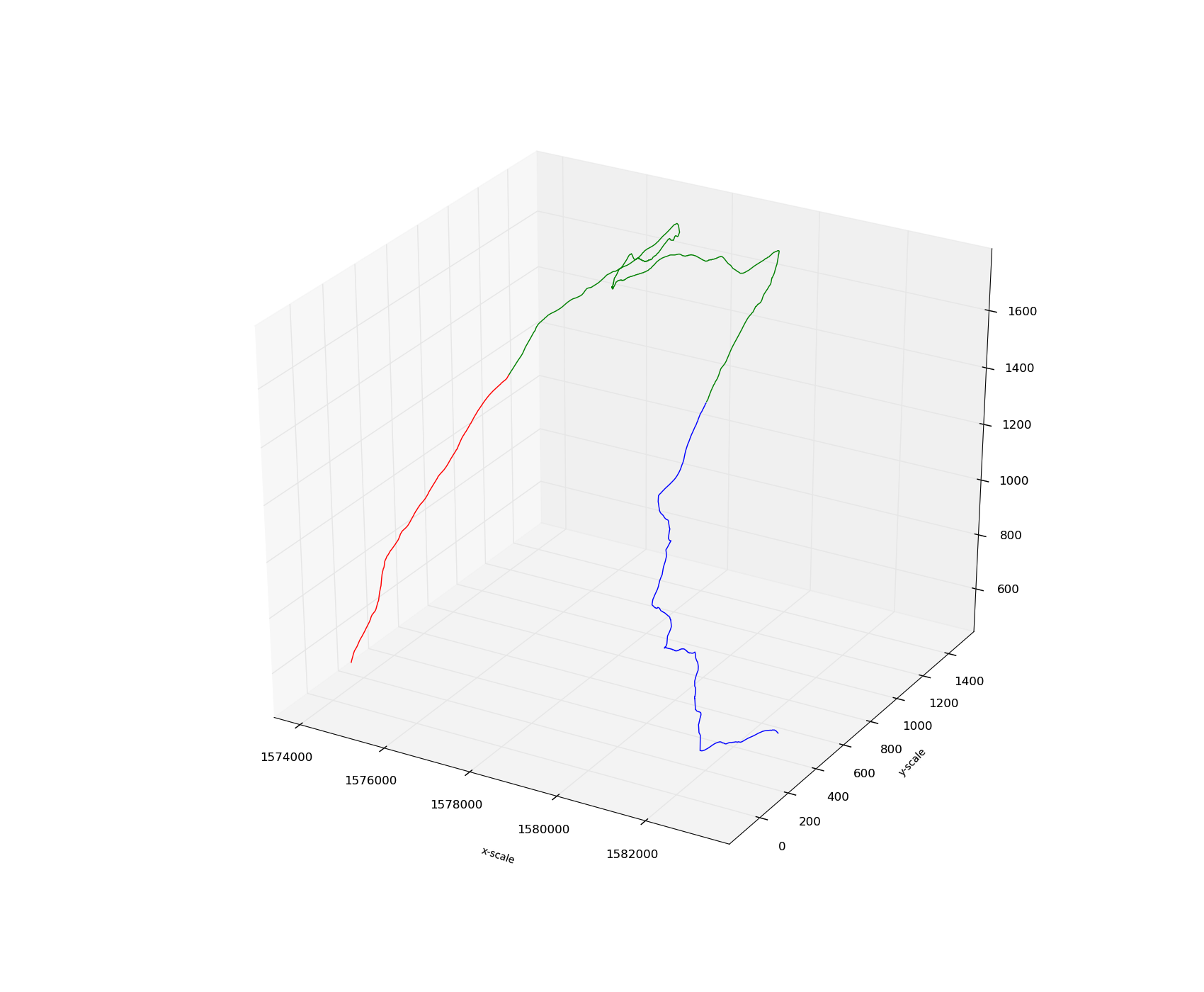# Problem plotting points in 3D

I am new to Matplotlib and am having some problems plotting the following set of coordinates (in python 2.6 and Win 7 32)
This is just a small sample of the data:
Seq.No. x-scale y-scale z-scale
01.000000 1579446.055280 5361974.495490 1342.967407
02.000000 1579446.646620 5361972.813700 1342.967407
03.000000 1579448.047050 5361968.830880 1341.237305
04.000000 1579450.992084 5361963.830880 1337.739502
05.000000 1579453.937117 5361958.830880 1336.262817

with the following outcome:
(plot3d.png)
Problem: while the x-scale is ok and the z-scale looks ok, the y-scale is definitely not ok. The numbers in the image are not the ones in the y-array (I double checked.)
I also have a number of questons:
Question1: how does one create a label for the z scale? (zlabel is not valid)
Queston2: Is it possible to fill below the line (so it looks like a mountain) and how
Question3: Is it possible to traverse sequentially all the points plotted in the image so as to make computations (such as distance to the next point, etc…).
The points themselves come from a text file but are not in sequence. They are sequenced by being plotted in their right position in 3D space.

This is the code I use to plot:

sx = np.array(x) #the x data extracted from a text file
sy = np.array(y) #the y data " "
sz = np.array(z) #the z data " "
Axes3D.plot(ax, sx, sy, sz)
xlabel(“x-scale”, horizontalalignment=‘right’, fontsize=‘small’, rotation=‘horizontal’)
ylabel(“y-scale”, horizontalalignment=‘right’, fontsize=‘small’, rotation=‘horizontal’)
savefig(“plot3d.png”)

GeorgeI am new to Matplotlib and am having some problems plotting the following set of coordinates (in python 2.6 and Win 7 32)
This is just a small sample of the data:
Seq.No. x-scale y-scale z-scale
01.000000 1579446.055280 5361974.495490 1342.967407
02.000000 1579446.646620 5361972.813700 1342.967407
03.000000 1579448.047050 5361968.830880 1341.237305
04.000000 1579450.992084 5361963.830880 1337.739502
05.000000 1579453.937117 5361958.830880 1336.262817

with the following outcome:
(plot3d.png)
Problem: while the x-scale is ok and the z-scale looks ok, the y-scale is definitely not ok. The numbers in the image are not the ones in the y-array (I double checked.)

George,

This is a known issue where very large axis values were being represented using an “offset” (much like in 2-d plots with very large axis values). The problem was that the offset was not displayed for 3d plots. This is definitely fixed in the latest development branch, but I can’t remember if I fixed it in the 1.0.1 release (probably not).

I also have a number of questons:
Question1: how does one create a label for the z scale? (zlabel is not valid)

This should be fixed by the next release. Most functions like set_xlim(), set_ylabel() and such merely call that function for the appropriate axis object. If a particular function is missing, you can call it yourself doing something like the following:

ax.zaxis.set_label()

Note that if you have an earlier version of matplotlib, you might have to do:

ax.w_zaxis.set_label()

Queston2: Is it possible to fill below the line (so it looks like a mountain) and how

Never tried considered anything like that. Might involve creating a 3D patch object with some sort of path completion. File a feature request on the matplotlib tracker here:

http://sourceforge.net/tracker2/?group_id=80706

Question3: Is it possible to traverse sequentially all the points plotted in the image so as to make computations (such as distance to the next point, etc…).
The points themselves come from a text file but are not in sequence. They are sequenced by being plotted in their right position in 3D space.

I am not exactly sure what you mean, but there is a nice data structure that I use to do efficient data operations on spatial data called KDTrees, which can be found in scipy:

http://docs.scipy.org/doc/scipy/reference/generated/scipy.spatial.KDTree.html